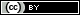Want to share your content on R-bloggers? click here if you have a blog, or here if you don't.

Many statistical modeling problems reduce to a minimization problem of the general form:

or

where $f$ is some type of loss function, $\mathbf{X}$ denotes the data, and $g$ is a penalty, also referred to by other names, such as “regularization term” (problems (1) and (2-3) are often equivalent by the way). Of course both, $f$ and $g$, may depend on further parameters.

There are multiple reasons why it can be helpful to check out the contours of such penalty functions $g$:

1. When $\boldsymbol{\beta}$ is two-dimensional, the solution of problem (2-3) can be found by simply taking a look at the contours of $f$ and $g$.
2. That builds intuition for what happens in more than two dimensions, and in other more general cases.
3. From a Bayesian point of view, problem (1) can often be interpreted as an MAP estimator, in which case the contours of $g$ are also contours of the prior distribution of $\boldsymbol{\beta}$.

Therefore, it is meaningful to visualize the set of points that $g$ maps onto the unit ball in $\mathbb{R}^2$, i.e., the set

Below you see GIF images of such sets $B\subscript{g}$ for various penalty functions $g$ in 2D, capturing the effect of varying certain parameters in $g$. The covered penalty functions include the family of $p$-norms, the elastic net penalty, the fused penalty, and the sorted $\ell_1$ norm.

:white_check_mark: R code to reproduce the GIFs is provided.

## p-norms in 2D

First we consider the $p$-norm,

with a varying parameter $p \in (0, \infty]$ (which actually isn’t a proper norm for $p < 1$). Many statistical methods, such as LASSO and Ridge Regression, employ $p$-norm penalties. To find all $\boldsymbol{\beta}$ on the boundary of the 2D unit $p$-norm ball, given $\beta_1$ (the first entry of $\boldsymbol{\beta}$), $\beta_2$ is easily obtained as## Elastic net penalty in 2D

The elastic net penalty can be written in the form

for $\alpha\in(0,1)$. It is quite popular with a variety of regression-based methods (such as the Elastic Net, of course). We obtain the corresponding 2D unit “ball”, by calculating $\beta\subscript{2}$ from a given $\beta\subscript{1}\in[-1,1]$ as## Fused penalty in 2D

The fused penalty can be written in the form

It encourages neighboring coefficients $\beta\subscript{i}$ to have similar values, and is utilized by the fused LASSO and similar methods.(Here I have simply evaluated the fused penalty function on a grid of points in $[-2,2]^2$, because figuring out equations in parametric form for the above polygons was too painful for my taste… :stuck_out_tongue:)

## Sorted L1 penalty in 2D

The Sorted $\ell\subscript{1}$ penalty is used in a number of regression-based methods, such as SLOPE and OSCAR. It has the form

where $\lvert \beta \rvert\subscript{(1)} \geq \lvert \beta \rvert\subscript{(2)} \geq \ldots \geq \lvert \beta \rvert\subscript{(m)}$ are the absolute values of the entries of $\boldsymbol{\beta}$ arranged in a decreasing order. In 2D this reduces to# Code

The R code uses the libraries dplyr for data manipulation, ggplot2 for generation of figures, and magick to combine the individual images into a GIF.

Here are the R scripts that can be used to reproduce the above GIFs:

Should I come across other interesting penalty functions that make sense in 2D, then I will add corresponding further visualizations to the same Github repository.